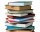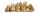# Examination 2

In an examination, a teacher awards 2 marks for every correct answer and subtract a mark for every wrong answer. If there are 20 questions and a child scored 25 marks how many correct answer did the child get?

Correct result:

c =  15

#### Solution:

25 = 2c - 1•(20-c)

25 = 2•c - 1•(20-c)

3c = 45

c = 15

Our simple equation calculator calculates it.We would be pleased if you find an error in the word problem, spelling mistakes, or inaccuracies and send it to us. Thank you!Tips to related online calculators
Do you have a linear equation or system of equations and looking for its solution? Or do you have quadratic equation?

## Next similar math problems:

• Unknown number 11That number increased by three equals three times itself?
• Find xSolve: if 2(x-1)=14, then x= (solve an equation with one unknown)
• Simple equation 1035= 7*3*x what is x?
• Simple equation 8Solve the following equation: 36=-(1+7x)-6(-7-x)
• Simple equation 9Solve the following equation: -8y+5=-9y+9
• Negative in equation2x + 3 + 7x = – 24, what is the value of x?
• Dropped sheetsThree consecutive sheets dropped from the book. The sum of the numbers on the pages of the dropped sheets is 273. What number has the last page of the dropped sheets?
• Equation 29Solve next equation: 2 ( 2x + 3 ) = 8 ( 1 - x) -5 ( x -2 )
• Simple equationSolve the following simple equation: 2. (4x + 3) = 2-5. (1-x)
• Simple equationSolve for x: 3(x + 2) = x - 18
• Write decimalsWrite in the decimal system the short and advanced form of these numbers: a) four thousand seventy-nine b) five hundred and one thousand six hundred and ten c) nine million twenty-six
• NormThree workers planted 3555 seedlings of tomatoes in one dey. First worked at the standard norm, the second planted 120 seedlings more and the third 135 seedlings more than the first worker. How many seedlings were standard norm?
• Forest nurseryIn the forest nursery after winter, they found that 1/10 stems died out of them. For them, they land 193 new spruces. How many spruces are in the forest nursery?
• Last pageTwo consecutive sheets dropped out of the book. The sum of the numbers on the sides of the dropped sheets is 154. What is the number of the last page of the dropped sheets?
• Number with onesThe first digit of the number is 1, if we move this digit to the end we get a 3 times higher number, which is the number?Readers borrowed a total of 220 books in the library during the first three days. On the second day, readers borrowed half as many books as the first day and at the same time 20 fewer books than the third day. Depending on the quantity x, express the numbTwenty rabbits are put in 4 cells so that there are different number of rabbits in each cell contains at least 3 rabbits. What is the largest possible number of rabbits in one cell